﻿ Molecular bond - hole in de Broglie wave

# What determines molecular bond ?

## Carbon in quantum mechanics is nonsense

### [ Carbon orbitals cannot be confirmed, just "imaginary" thing ]

(Fig.1)  Carbon with 4 valence electrons (= 2 electrons in 2s, 1+1 in 2p ? )Carbon (= C ) has 4 valence electrons.  According to quantum mechanics, Carbon's 2 electrons in 2s orbital and 1 electron in each 2p orbital.

The point is these quantum orbitals cannot be seen, so they are just "imaginary" things.  Electron spin is faster-than-light, lacking reality.

They claim each orbital can have up to two electrons of spin up and down.  But too weak spin disagrees with Pauli exclusion principle.

So this Cabon model by quantum mechanics is unreal and inconsistent.  We need realistic and consistent atomic model !

## Carbon in quantum mechanics is contradictory

### [ Carbon's 4 valence electrons are "tetrahedrally" distributed ]

(Fig.2)  Then, original Carbon's orbital model (= 2s, 2p ) is wrong ?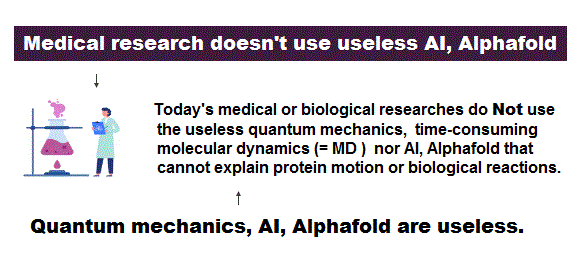As shown in methane (= CH4 ), Carbon's 4 valence electrons are observed to be tetrahedrally distributed in experiments.

Considering the symmetric Coulomb repulsion, this "tetrahedral distrubution" of Carbon valence electrons is quite natural and realistic.

This means the original quantum mechanical orbitals are meaningless an useless.  They artificially changed the original definition of orbitals to fit experimental results.

So quantum mechanical model using orbitals and spin is self-contradictory.  We need more realistic atomic model.

## Quantum spin model is false

### [ Electrons spin up + down → molecular bond ? ]

(Fig.3)  ↓ This spin up, down molecule model disagrees with actual strengthAccording to quantum mechanics, electron spins up and down form each molecular bond such as H2.  But of course, these unrealistic spins cannot be seen.

Furthermore, spin-spin magnetic interaction is too weak to explain actual molecular bond strength, so these spin model is unrealistic and wrong.

## Up, down spins really form molecular bond ?

### [ Electron "spin" is unreal, too weak to form atomic bond ! ]

(Fig.4)  Unreal spin is really involved in hydrogen molecule bond ?Textbooks in quantum mechanics often argue that "up" and "down" spins form molecular bonds.  But in fact it's physically impossible.

Because spin-spin magnetic interaction (= only 0.001 eV ) is too weak to cause any molecular bonds (= 3-4 eV ).  So spin has nothing to do with molecular bonds !

## Electron spinning far exceeds light speed !

### [ Spinning speed of "point"-like electron is much faster than light ! ]

(Fig.5)  Point-like electron ( radius r → 0 ), rotation v → ∞Angular momentum is given by mv × r ( v = velocity, r = radius ).
Electron spin also has angular momentum 1/2ħ, they claim

The problem is an electron is very tiny, point-like.
The point-like particle means its radius r is almost zero.

So to get the angular momentum 1/2ħ, the electron spinning must far exceed light speed ( this p.5, this )

So the electron spin lacks reality.
Even Pauli ridiculed the idea of "spinning electron".

But in "s" orbital of Schrodinger's hydrogen, this electron spin is the only generator of magnetic moment.

So they had no choice but to accept this strange spin ( Not as real spinning and speed ).

## Electron spin is too weak to form molecular bond !

### [ Spin magnetic enrgy is far smaller than bond energy ! ]

(Fig.6)  Spin-spin magnetic energy (= 0.0001 eV ) vs. bond energy (= 4.5 eV )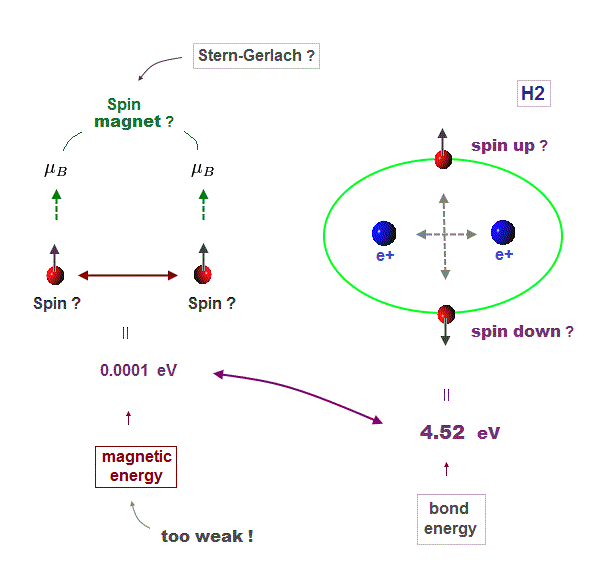You may hear spin is tiny magnet with the magnitude of Bohr magneton.
We cannot see unreal spin itself, we just measure its magnetism by experiments.

We can replace unrealistic spin by real orbital motion causing the same magnetic field.  "Spin" cannot explain actual phenomena.

Spin-spin magnetic interaction is too weak to explain actual ferromagnet.
See this p.7, this p.6.  So spin model failed from the beginning.

Hydrogen molecular bond energy is known to be as large as 4.5 eV which electrons spin's magnetic energy (= only 0.0001 eV ! ) can NEVER reach.

## Neon -- 2 × de Broglie wavelength

(Fig.7)  2 × de Broglie wavelength orbits. Each is a pair of opposite phases.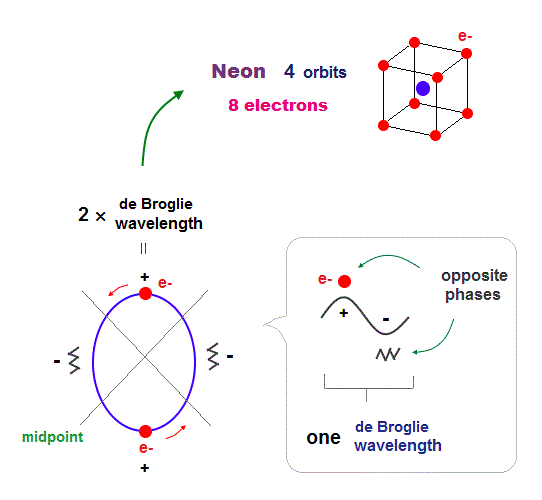On this page, we explain Neon has 4 orbits with 2 × de Broglie wavelength.

One wavelength consists of a pair of "crest" (= + ) and "trough" (= - ) irrespective of transverse and longitudinal waves.

Here we suppose "+" phase contains an electron itself, and "-" phase is compressed by the electron's movement,   --- which is " de Broglie wave ".

2 × ( 1 × ) de Broglie wavelength orbit contains two ( one ) pairs of ±opposite phases and two ( one ) midpoint lines.

These "opposite" wave phases cancel each other by destructive interference.
To avoid it, two orbits must cross perpendicularly in all atoms.

## 2 × de Broglie wavelength → maximum 4 orbits

### [ Neon consists of 8 electrons in 4 orbits with 2 × wavelength. ]

(Fig.8)  All opposite wave phases just cross each other in Neon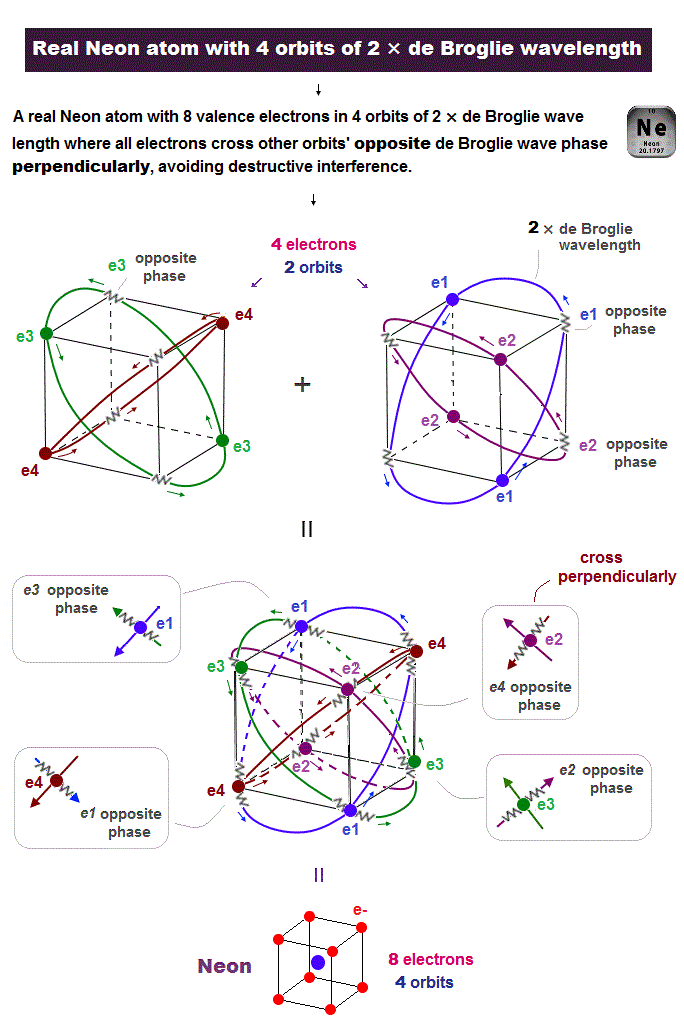Neon contains eight valence electrons.  Neon belongs to the 2nd line in periodic table, meaning its orbit is 2 × de Broglie wavelength.

When just two 2 × de Broglie wavelenght orbits cross each other (= Fig.8 upper ), some electrons are free from their opposite wave phases.

With four orbits, all electrons and their opposite wave phases cross each other, forming stable noble gas Neon with no more room.

## Carbon includes four pairs of electron and "hole"

### [ Carbon's four valence electrons form "tetrahedral" structure. ]

(Fig.9)  Carbon (= C ) is 4 electrons and "holes" in 4 orbits.When each 2 × de Broglie wavelength orbit includes one electron and one hole, it generates four "holes".  This is carbon (= C ) atom.

Carbon consisting of four valence electrons form tetrahedral structure with bond angle 109.5o.

## Carbon structure and de Broglie wave.

### [ Each electron ( hole ) crosses the opposite de Broglie wave phase ]

(Fig.10)  4 valence electrons and 4 holes in Carbon (= C ).Carbon has 4 valence electrons in four 2 × de Broglie wavelength orbits.  So each orbit consists of 1 electron and 1 hole

When the opposite phases of de Broglie wave overlap, they cancel each other by destructive interference.

To avoid its cancellation, each electron ( and hole ) must cross the opposite wave phase perpendicularly.

## Carbon - carbon bond is formed by electron-hole pair

(Fig.11)  In C-C bond, an electron synchronize with another hole.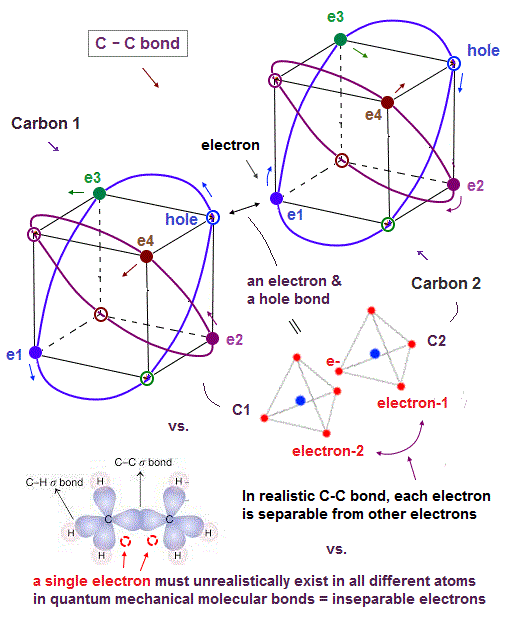Without unrealistic spins, how is each molecular bond formed ?   Considering Coloumb repulsion among electrons, an electron tends to approach "vacant hole".

Also in carbon-carbon ( C-C ) bond, electons are thought to aim at stable hexahedral structure like Neon by electron-hole pairs.

Furthermore, for each electron to keep stable, an electron has to enter the place of the "same wave phase".  A vacant hole meets this condition, too.

## The "Same" wave phases overlap in molecular bond

### [ Carbon 1's hole and Carbon 2's electron overlap to form C-C bond ! ]

(Fig.12)  Carbon 1's electron and Carbon 2's hole have the "same phase", so OKOne de Broglie wavelength consists of a pair of opposite phases where an electron ( or hole ) phase and its antiphase exist.

To form C-C molecular bond, an electron in Carbon 2 needs to enter the place of its same phase (= "hole" ) in Carbon 1.

By forming "electron-hole pair" in each molecular bond, their de Broglie wave phases avoid destructive interference, and its binding becomes stable.

In each bonding, the opposite wave (anti) phase crosses electron's de Broglie wave "perpendicularly" to avoid destructive interference.

## Electron cannot bind to places other than "hole" !

### [ Because there are no more space for electron's phase in each orbit. ]

(Fig.13)  Carbon = 2 × de Broglie wavelength orbit. A hole is the only binding placeCarbon has 4 valance electrons and can form up to 4 molecular bonds.  What determines the maximum binding number (= 4 bonds in Carbon molecule ) ?

Electrons' spin-spin magnetic interaction is too weak, so has almost NO influence on each molecular bond.  So molecular model based on spin is meaningless.

Only "de Broglie wave's interference" is left to explain molecular bond rule.  If an electron can bind only to the "hole", Carbon's 4 molecular bonds can be explained !

Because Carbon has 4 holes.  So the maximum molecular bond (= electron - hole pair ) number is 4.  Then why each electron cannot bind to places other than a hole ?

If an electron tries to enter the place other than an hole, it has to create "new electron's phase space" in that entry (= binding ) place.

But each Carbon orbit is 2 × de Broglie wavelength, so there are NO more space for creating new electron's phase.  This de Broglie wave interferernce determines molecular bond.2016/10/14 updated. Feel free to link to this site.